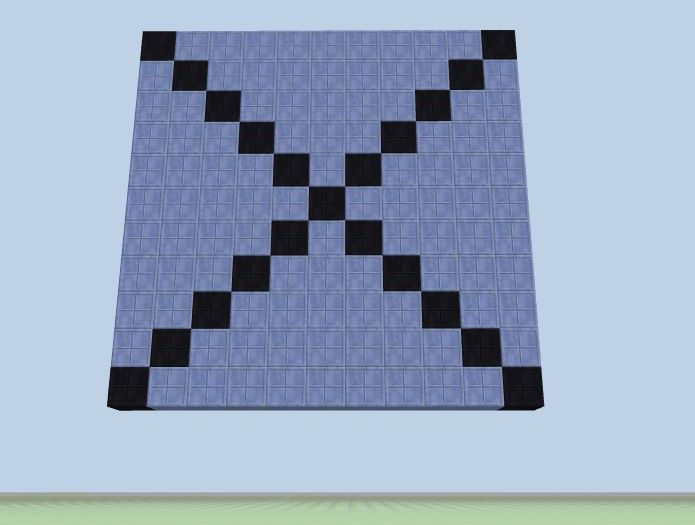# Flow of Control

`if/else if/else` statements are used to make complex decisions. It's perhaps the most used statement to control program flow.

Let's input a choice, then see the visual result in CodeCraft world:

### Example: `choice`

Let's build 5 white columns, then depending on your choice, one of them will be highlighted with a specific color:

``````// the original number array, white columns
let pool = [3, 2, 4, 8, 1];
for (let i in pool) {
column(i, -20, pool[i], 'quartz');
}
``````

Run to see the original 5 white columns, then add the following code:

``````let choice = Number(prompt('Enter your choice of number from 1 to 5: '));

if (choice == 1) {
console.log( '1, blue' );
column(0, -20, pool[choice-1], 'linen_blue');
}
else if (choice == 2) {
console.log( '2, red' );
column(1, -20, pool[choice-1], 'linen_red');
}
else if (choice == 3) {
console.log( '3, yellow' );
column(2, -20, pool[choice-1], 'linen_yellow');
}
else if (choice == 4) {
console.log( '4, pink' );
column(3, -20, pool[choice-1], 'linen_pink');
}
else if (choice == 5) {
console.log( '5, purple' );
column(4, -20, pool[choice-1], 'linen_purple');
}
else {
console.log('You entered an invalid choice. No column is chosen');
}
``````

Run the whole program a few times. Each time enter a different number to see the chosen column highlighted with a color.

Such as: `4, pink`### Extra Practice

Let's make a square that is a solid color but has a different color on its diagonals. This can be done with a ternary operation inside nested `for` loops:

##### Square_X
``````for (let i = -5; i < 6; i++) {
for (let j = -5; j < 6; j++) {
let m = (Math.abs(i) == Math.abs(j)) ? 'box_black' : 'box_blue';
block(i, 9+j, -10, m);
}
}
``````